Next: Multi-Particle Systems Up: Simple Harmonic Oscillator Previous: Simple Harmonic Oscillator

### Exercises

1. Show that the wavefunction of a particle of massin an infinite one-dimensional square-well of widthreturns to its original form after a quantum revival time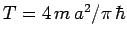.

2. A particle of massmoves freely in one dimension between impenetrable walls located at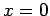and. Its initial wavefunction is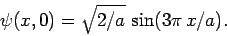What is the subsequent time evolution of the wavefunction? Suppose that the initial wavefunction is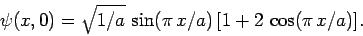What now is the subsequent time evolution? Calculate the probability of finding the particle between 0 andas a function of time in each case.

3. A particle of massis in the ground-state of an infinite one-dimensional square-well of width. Suddenly the well expands to twice its original size, as the right wall moves fromto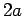, leaving the wavefunction momentarily undisturbed. The energy of the particle is now measured. What is the most probable result? What is the probability of obtaining this result? What is the next most probable result, and what is its probability of occurrence? What is the expectation value of the energy?

4. A stream of particles of massand energy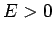encounter a potential step of height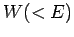: i.e.,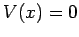for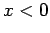andforwith the particles incident from. Show that the fraction reflected is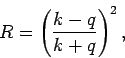whereand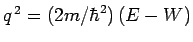.

5. A stream of particles of massand energyencounter the delta-function potential, where. Show that the fraction reflected iswhere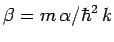, and. Does such a potential have a bound state? If so, what is its energy?

6. Two potential wells of widthare separated by a distance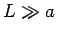. A particle of massand energyis in one of the wells. Estimate the time required for the particle to tunnel to the other well.

7. Consider the half-infinite potential well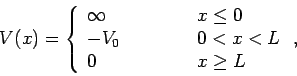where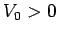. Demonstrate that the bound-states of a particle of massand energy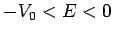satisfy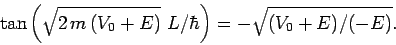8. Find the properly normalized first two excited energy eigenstates of the harmonic oscillator, as well as the expectation value of the potential energy in theth energy eigenstate. Hint: Consider the raising and lowering operatorsdefined in Eq. (408).Next: Multi-Particle Systems Up: Simple Harmonic Oscillator Previous: Simple Harmonic Oscillator
Richard Fitzpatrick 2010-07-20